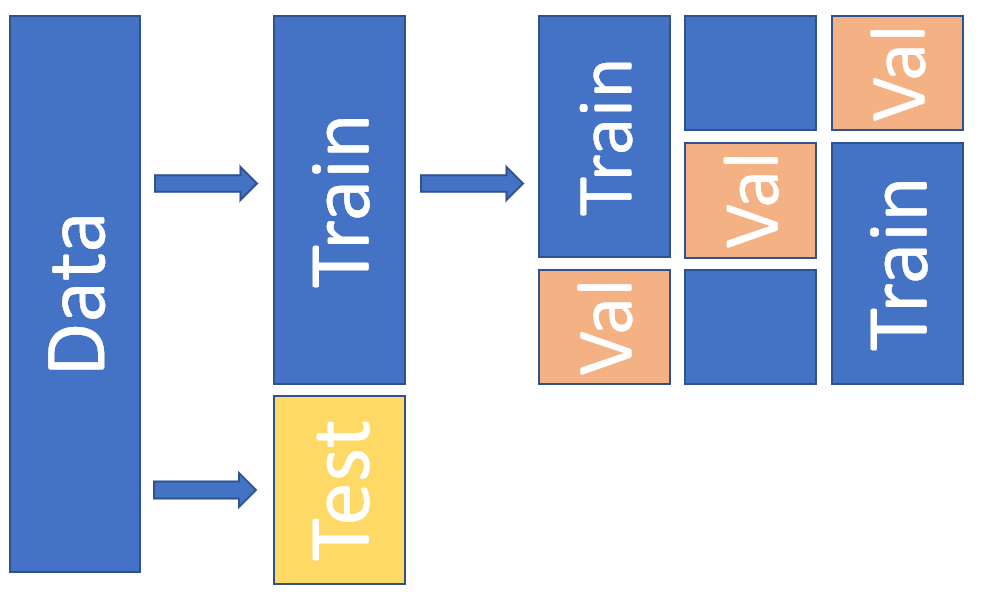# How to trust AI — a brief explanation about Cross Validation (and a little bit about model…

Original article was published on Deep Learning on Medium# How to trust AI — a brief explanation about Cross Validation (and a little bit about model interpretability)

When I explain machine learning-based AI applications or give a lecture in the conference, I often get questions from the audience, “I understand that AI learns data, but why can we trust the values AI gives us to be correct?” “What is the way to make sure AI is giving the correct answer?”

In other words, the question is how do we check the accuracy of the learned AI? This is important, and if you blindly trust that you’ve made them learn from the data, they may still be in a state of “overfitting,” and they may not work well with actual production data or in the actual environment.

In order to trust that the AI is correct, we usually use a technique called “cross-validation”.

In machine learning-based AI, there is always an error between the true correct answer and the one guessed by the AI, and we try to make the error as small as possible.

When you learn, you will have data and a set of correct answers to it. We will train the AI to know that the data and the correct answer correspond to each other. Practically, in this case, the data and the correct answer set are divided into three. It’s data for training, data for validation, and data for testing.

First, the AI learns using the training data. It learns that the correct answer A corresponds to data X, etc.

Then, the learned AI will return what seems to be the correct answer to the data. Next, it will use the validation data to validate whether trained AI with this approach is enough good or not. In some cases, the validation would show the AI model should be modified or the initial values (hyperparameters) should be adjusted in order to reduce the error.

Finally, we use the test data to see how close the output AI returns is to the correct answer.

This whole process is cross-validation.

The reason why there are two types of data, validation data and test data, is because we are using the validation data to adjust the model, so if there is a bias in the validation data, or if it is overly adjusted (over-learned), it is a problem. Therefore, at the end, we evaluate how many correct answers the AI returns using separately prepared test data that we do not use to modify the model, but just test. Ideally, it would fit the data for training/validation (= less model bias) and fit the data for testing (= less model variance), but this is a troubling trade-off relationship.

The basic way to trust AI is to gain confidence in the AI based on the percentage of correct answers to this test data. This is because many of the current mainstream AI technologies (e.g., ensemble learning, reinforcement learning, deep learning, etc.) have a black box of learning results and it is difficult to fully and clearly confirm the internal processing of how the answer was computed. Recently, however, there have been approaches to estimate the contribution of learning some data to AI results, so that the AI’s learning model can be modified. For instance, here’s a video that briefly introduces LIME, an approach that’s been getting a lot of attention lately.